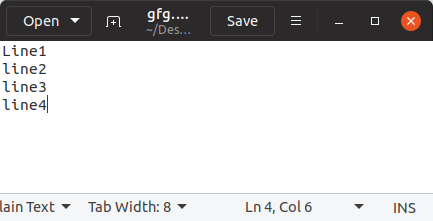# Count number of lines in a text file in Python

Counting the number of characters is important because almost all the text boxes that rely on user input have a certain limit on the number of characters that can be inserted. For example, If the file is small, you can use readlines() or a loop approach in Python.

```Input: line 1
line 2
line 3
Output: 3
Explanation: The Input has 3 number of line in it so we get 3 as our output.```

## Count the Number of Lines in a Text File

• Using enumerate
• Use Loop and Counter to Count Lines
• Use the Loop and Sum functions to Count Lines

Below is the implementation.

Let’s suppose the text file looks like thismyfile.txt

### Python counts the number of lines in a string using readlines()

The readlines() are used to read all the lines in a single go and then return them as each line is a string element in a list. This function can be used for small files.

## Python3

 `with ``open``(r``"myfile.txt"``, ``'r'``) as fp:` `    ``lines ``=` `len``(fp.readlines())` `    ``print``(``'Total Number of lines:'``, lines)`

Output:

`Total Number of lines: 5`

Time complexity: O(n).
Auxiliary space: O(1).

### Python counts the number of lines in a text file using enumerate

Enumerate() method adds a counter to an iterable and returns it in the form of an enumerating object.

## Python3

 `with ``open``(r``"myfile.txt"``, ``'r'``) as fp:` `    ``for` `count, line ``in` `enumerate``(fp):` `        ``pass` `print``(``'Total Number of lines:'``, count ``+` `1``)`

Output:

`Total Number of lines: 5`

Time complexity: O(n), where n is the number of lines in the text file
Auxiliary space: O(1)

### Use Loop and Counter to Count Lines in Python

Loops Python is used for sequential traversal. here we will count the number of lines using the loop and if statement. if help us to determine if is there any character or not, if there will be any character present the IF condition returns true and the counter will increment.

## Python3

 `file` `=` `open``(``"gfg.txt"``, ``"r"``)` `Counter ``=` `0`   `# Reading from file` `Content ``=` `file``.read()` `CoList ``=` `Content.split(``"\n"``)`   `for` `i ``in` `CoList:` `    ``if` `i:` `        ``Counter ``+``=` `1`   `print``(``"This is the number of lines in the file"``)` `print``(Counter)`

Output:

```This is the number of lines in the file
4```

### Use the Loop and Sum functions to Count Lines in Python

Loops Python is used for sequential traversal. here we will count the number of lines using the sum function in Python. if help us to determine if is there any character or not, if there will be any character present the IF condition returns true and the counter will increment.

## Python3

 `with ``open``(r``"myfile.txt"``, ``'r'``) as fp:` `    ``lines ``=` `sum``(``1` `for` `line ``in` `fp)` `    ``print``(``'Total Number of lines:'``, lines)`

Output:

`Total Number of lines: 5`

Time complexity: O(n), where n is the number of lines in the text file
Auxiliary space: O(1)

Whether you're preparing for your first job interview or aiming to upskill in this ever-evolving tech landscape, GeeksforGeeks Courses are your key to success. We provide top-quality content at affordable prices, all geared towards accelerating your growth in a time-bound manner. Join the millions we've already empowered, and we're here to do the same for you. Don't miss out - check it out now!

Previous
Next